Reach Us+44-1625-708989
Enhanced Technique via Filters for Seizure Prediction | OMICS International
Journal of Bioengineering & Biomedical Science

Like us on:

# Enhanced Technique via Filters for Seizure Prediction

Ahmed S*, El-Khobby H, Mahmoud A and Abd El-Samie FE

Electronics and Communications Department, Faculty of Engineering, Tanta University, Egypt

*Corresponding Author:
Ahmed S
Electronics and Communications Department
Faculty of Engineering, Tanta University, Egypt
Tel: +201226521508
E-mail: [email protected]u.eg

Received date: December 07, 2016; Accepted date: February 28, 2017; Published date: March 05, 2017

Citation: Ahmed S, El-Khobby H, Mahmoud A, El-Samie AFE (2017) Enhanced Technique via Filters for Seizure Prediction. J Bioengineer & Biomedical Sci 7:218. doi: 10.4172/2155-9538.1000218

Copyright: © 2017 Ahmed S, et al. This is an open-access article distributed under the terms of the Creative Commons Attribution License, which permits unrestricted use, distribution, and reproduction in any medium, provided the original author and source are credited.

Visit for more related articles at Journal of Bioengineering & Biomedical Science

#### Abstract

This research study reports on the effective band of EEG signal to be used in seizure prediction, such as gamma, beta, alpha etc. The exercises were performed on a patient-specific framework for Electroencephalography (EEG) channel selection and seizure prediction, based on statistical probability distributions of the EEG signals. This framework is an enhanced method consists of two major phases, training and testing. Our objective was to distinguish between predicted and normal EEG signals. We achieved high prediction efficiency in reasonable time with low false alarm rate considering the parameters of seizure prediction techniques. Overall, we reached an efficiency of 96.2485% with prediction time of 54.012 min and false alarm rate of 0.10526/h. This approach is having considerable significance. It is a simple method which depends on all filtering technique. This method can be implemented easily in future work and it doesn’t have much computational load.

#### Keywords

EEG; Seizure prediction; Channel selection; Statistical analysis; EEG decomposition; Elliptic filter

#### Introduction

Berger et al.  inspired to continue exploration in EEG signal processing and associated direction. EEG signal processing has several applications either as medical applications such as seizure detection and prediction [2,3] or non-medical applications including games and safety [4,5]. Epilepsy-related studies are the most interesting among these applications. Around 1% of the world population suffers from epilepsy and it is accompanied by seizures that can be traced by EEG . The objective of this study was seizure prediction in EEG signals with suitable prediction time. Early anticipation of seizures can be used to alert to save patients from dangers. The efficiency of a seizure prediction algorithm is estimated with the prediction rate, false alarm rate, and prediction horizon [7-13].

Various researchers have investigated EEG seizure prediction using different techniques. Some of these efforts are tabulated in Table 1.

Author Method Sensitivity/Efficiency False alarm rate
Parvez M and Paul M  Customized phase correlation 95% 2.45 per patient
Arabi  Entropy 90.20% 0.11/h
Shufang Li et al.  Spike rate of interactional EEG 75.80% 0.09/h
Wang et al.  Reinforcement learning with online monitoring. 70% ------
Khalid MI et al.  LLE for EEG classification 88% ----
Hung et al.  Wavelet transform 87% 0.24/h
Gadhoumi et al.  Wavelet with measuring the similarity with a reference EEG signal 85% 0.35/h

Table 1: Some efforts of seizure prediction.

Empirical mode decomposition had been used in seizure prediction. For example, Qi et al. used EEG empirical mode decomposition (EMD) for seizure prediction with different prediction horizons . They achieved a high sensitivity with time delays of 10-20 seconds. In addition, Principal Component Analysis (PCA) has been used as a pre-processing signal separation step for robust seizure prediction algorithms [15-17]. The main disadvantage of EMD is computation latency.

This paper presents a new time-domain approach for EEG channel selection and hence seizure prediction based on simple statistics with the efficient frequency band. The main idea was to discriminate between different signal activities based on their PDFs. Simulation experiments have proved that if non-overlapping segmentations of signals are traced, difference occurs to PDFs of segments even for the same category of segments. This means, PDF bins treatment could be made as random variables and selection of the most appropriate bins for discrimination can be done through a simple threshold process. The following section of the article gives a detailed explanation of the proposed approach followed by details of the pre-processing steps implemented prior to PDF calculation, EEG sub-banding and simulation results.

#### Proposed Seizure Prediction Approach

The proposed channel selection and seizure prediction approach depend mainly on estimating the PDFs of signal amplitude, derivative, local mean, local variance, and median of the different signal channels (Figure 1). Our approach consists of two phases; training and testing as illustrated (Figure 2). In the training phase, few hours were randomly selected for normal activities and two or three intervals for ictal and preictal activities. The selected periods with multi-channels are segmented into 10-second segments. Five PDFs were estimated, for each channel in each segment, (amplitude, derivative, local mean, local variance, and median).

Figure 2: Training and testing phases of the proposed channel selection and seizure prediction algorithm.

Treatment for each PDF bin (9 bins) was done as a random variable for each of the normal, ictal, pre-ictal histogram classes. Based on predefined false alarm and prediction probability thresholds, the bins and the channels that discriminate between normal and pre-ictal classes were selected for discrimination in the testing phase.

#### Pre-Processing of EEG Signals

The proposed method depends on many pre-processing operations performed on the signal channels such as the derivative, local mean, local variance, and median. The derivative of an EEG signal reinforces the rapid transitions in the signals and degrades slow transitions. Hence, the different activities of the signal will yield distinguishable derivatives through their PDFs. The local mean is a good indication of the signal trend, and the local variance characterizes the signal power very well from sample to sample. The median filtering process removes any spikes that may have resulted from impulsive noise during the signal recording process. Based on the five estimated PDFs signal amplitude; derivative, local mean, local variance, and median for any EEG segment, discrimination can be done between normal, ictal, and pre-ictal signal segments.

Signal derivative

Abnormal activities in EEG signal are accompanied by sudden changes in amplitude. To reinforce these sudden changes, a signal differentiator can be utilized. We used a digital first-order differentiator filter for this purpose. This filter is given by :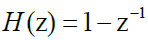Local mean

Local mean can be estimated for a signal X (m) as follows :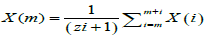(2)

While (2i+1) refers to the number of samples in the used segment for the estimation.

Local variance

Local variance can be estimated for a signal X (m) as follows :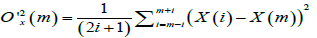(3)

#### Median Filtering

Median filtering is a sort of nonlinear smoothing operation of signals. It reduces some of the spikes in signals that may occur due to impulse noise. In the median filtering process, an odd set of signal samples were sorted. The middle value after sorting was extracted. For a median filter of length N=2i+1, the filter output is given as :

Y(m) = MED[ X(m-i),....,X(m),….,X(m+i) ]   (4)

While, X (m) and Y (m) are the samples of the input and output sequences, respectively.

This type of median filtering is non-recursive so that an estimation of median filter output at any time is independent of the other results. Another type of median filtering is recursive. For a recursive median filter with window length N = 2K +1, the output is defined as :

Y(m) = MED[ Y(m-i),Y(m-i+1)….,Y(m-1),X(m),….,X(m+i) ]   (5)

This recursion process is a type of feedback that reduces noise more efficiently.

#### Results

Simulation experiments were carried out on 5 patients from MIT database (patients 1, 8, 11, 14, 20) with 148.6133 hours containing 31 seizures for better understanding and assessment of the steps of the proposed approach .

Our main objective was to show the effective band of EEG signal that results in the best way to distinguish between normal and pre-ictal activities for early seizure prediction. We used the elliptic digital filter as a powerful filtering tool and display results. Elliptic digital band-pass filter, with order K=20 and bandwidth BW from 0 to 30 Hz was used.

A moving average filter was used to refine the results (Figure 3), because a decision of a certain activity was not taken with a single signal segment. Multiple segments were required in the decision. Hence, the moving average process was appropriate for this action. The discrimination count on the vertical axis was compared with a selected threshold to determine the pre-ictal regions.

Figure 3: Variation of the discrimination count with time for the selected five patients with adding S-G digital smoothing filter.

In the simulation experiments, we tested three different prediction horizons of 30, 60, and 90 minutes. In addition, a 15-minute postseizure horizon was adopted during the interpretation of the results. The obtained results for 5 MIT patients are tabulated in Table 2.

Patient No. and data 30 Minutes horizon 60 Minutes horizon 90 Minutes horizon
Patent No. 1
Total No. of hours=40.5522 hours
Total No. of seizures=7
Pred. prob. constraint=70%
False alarm prob. constraint=30%
Filter length=60
Frequency band=0-30 Hz
Decision threshold= 7 5
No. of predicted seizures= 5
No. of false alarms= 8
Average prediction time= (25.933333+30+30+27.2833333+29.3337)/5= 28.51 min
No. of predicted seizures= 6
No. of false alarms= 6
Average prediction time= (25.933333+58.95+37.083333+43.5+27.28333+29.39997)/6= 37.02499 min)
No. of predicted seizures= 6
No. of false alarms= 5
Average prediction time= (66.26667+90+37.0833333+43.5+27.28333+29.36667)/6= 48.91667 min
Patent No. 8
Total No. of hours= 20 hours
Total No. of seizures=5
Pred. prob. constraint=70%
False alarm prob. constraint=30%
Filter length=60
Frequency band=0-30 Hz
Decision threshold = 26
No. of predicted seizures= 3
No. of false alarms= 3
Average prediction time (0+16.76667+30)/3= 15.58889 min
No. of predicted seizures=5
No. of false alarms=2
Average prediction time (34.5+16.76667+46.3+55.11667+42.71667)/5= 39.08 min
No. of predicted seizures=5
No. of false alarms=2
Average prediction time= (34.5+16.76667+46.3+55.11667+42.71667)/5= 39.08 min
Patent No. 14
Total No. of hours= 25.851 hours
Total No. of seizures=8
Pred. prob. constraint=60%
False alarm prob. constraint=40%
Filter length=60
Frequency band=0-30 Hz
Decision threshold=5
No. of predicted seizures = 8
No. of false alarms= 7
Average prediction time= (17.1+30+30+30+30+0.316667+23.65+18.55)/8 = 22.45208 min
No. of predicted seizures = 8
No. of false alarms= 5
Average prediction time= (58.433337+60+60+60+36.3+0.316667+23.65+36.71667)/8 = 41.92708 min
No. of predicted seizures = 8
No. of false alarms= 5
Average prediction time= (76.433333+64.2+88.283333+90+90+0.316667+23.65+36.71667)/8 = 58.7 min
Patent No. 20
Total No. of hours=27.595 hours
Total No. of seizures=8
Pred. prob. constraint=70%
False alarm prob. constraint=30%
Filter length=60
Frequency band=0-30 Hz
Decision threshold=10 0
No. of predicted seizures = 6
No. of false alarms=0
Average prediction time= (15.4+12.833333+30+30+10.48333+13.3333333)/6 = 18.675 min
No. of predicted seizures = 8
No. of false alarms=0
Average prediction time= (15.4+12.833333+30.466667+56.01667+30+44.4833333+48.433333+48.21667)/8 = 35.73125 min
No. of predicted seizures = 8
No. of false alarms=0
Average prediction time= (15.4+90+87.46667+81.683333+83.81667+44.4833333+79.43333+72.55)/8 = 69.3517 min
Total No. of Seizures=28
Total No. of hours=
113.9982
Total No. of predicted seizures=22
Prediction rate=78.5714%
Total No. of false alarms=18
False alarm rate=0.1578/h
Average prediction time=21.30649 min
Total No. of predicted seizures= 27
Prediction rate = 96.2485%
Total No. of false alarms= 13
False alarm rate=0.114/h
Average prediction time= 38.44083 min
Total No. of predicted seizures= 27
Prediction rate = 96.2485%
Total No. of false alarms= 12
False alarm rate= 0.10526/h
Average prediction time =54.012 min

Table 2: Summary of the prediction results.

#### Discussion

This paper shows the effect of adding an elliptic digital filter to the proposed channel selection approach as discussed previously. At this juncture, the objective was to calculate the seizure prediction parameters. These parameters were prediction time, false alarm rate and efficiency.

Prediction time is the time that system make an alarm to the patient that a predicted seizure would occur. It can be calculated as: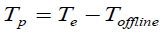(7)

Where Tp is the prediction time, Te is the estimated prediction time due to the selected threshold and Toffline is the offline seizure time from the MIT database.

False alarm rate is the rate that system makes an alarm for a seizure and wouldn’t occur. This false alarm can be calculated as: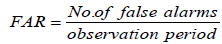(8)

The efficiency of proposed approach is the percentage of the predicted seizures overall observed seizures.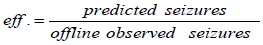(9)

These parameters are shown in the Table 2 with their respective values.

A brief comparison is shown in the Table 3 that shows we got an efficiency of 96.2485% which is comparatively better than previous efforts with suitable false alarm rate of 0.10526/h. In addition, we achieved prediction time of 54.012 min which is suitable to generate the alarm for the patient and predict the seizure case in advance.

Author Method Sensitivity/Efficiency False alarm rate
Parvez M and Paul M  Customized phase correlation 95% 2.45 per patient
Arabi  Entropy 90.2% 0.11/h
Shufang Li et al.  Spike rate of interactional EEG 75.8% 0.09/h
Wang et al.  Reinforcement learning with online monitoring. 70% ------
Khalid MI et al.  LLE for EEG classification 88% ----
Hung et al.  Wavelet transform 87% 0.24/h
Gadhoumi et al.  Wavelet with measuring the similarity with a reference EEG signal 85% 0.35/h
Our work Statistical channel selection with adding digital filters 96.2485% 0.10526/h

Table 3: Comparison between our work and previous efforts.

#### Conclusion

This paper presented an effective band of EEG signal with a statistical time-domain approach for EEG channel selection and seizure prediction depending on the estimation of the PDFs of the signals and the pre-processed versions. This approach is of multi-channel nature and it depends on pre-defined constraints for the required prediction and false alarm probabilities. Decision fusion and moving average post processing steps are utilized to enhance the prediction time and to achieve high prediction accuracies. The proposed method was utilized for different prediction horizons. It achieved prediction rates of 78.5714%, 96.2485%, and 96.2485% respectively for prediction horizons of 30, 60, and 90 minutes, with corresponding false alarm rates of 0.1578/h, 0.114/h, and 0.10526/h. The average prediction times were 21.30649 min, 38.44083 min, and 54.012 min for the 30, 60, and 90 minutes horizons, respectively. These obtained results show that the proposed prediction method can be efficiently used for long prediction horizons.

#### Compliance with Ethical Standards

Conflict of interest

Ahmed Sedik, Heba El-Khobby, Mahmoud Atea, and Fathi Abd El-Samie declare that no conflict of interest.

Ethical approval

This article does not contain any studies with human participants or animals performed by any of the authors.

#### References

Select your language of interest to view the total content in your interested language

### Article Usage

• Total views: 1775
• [From(publication date):
March-2017 - Mar 23, 2019]
• Breakdown by view type
• HTML page views : 1644

## Post your commentCan't read the image? click here to refresh
###### Peer Reviewed Journals

Make the best use of Scientific Research and information from our 700 + peer reviewed, Open Access Journals

International Conferences 2019-20

Meet Inspiring Speakers and Experts at our 3000+ Global Annual Meetings

Top